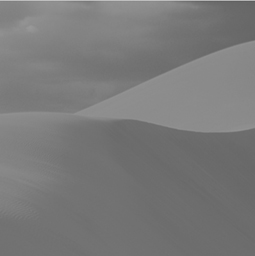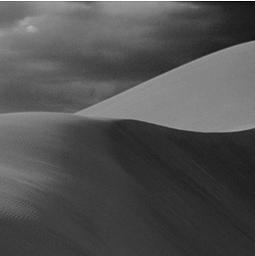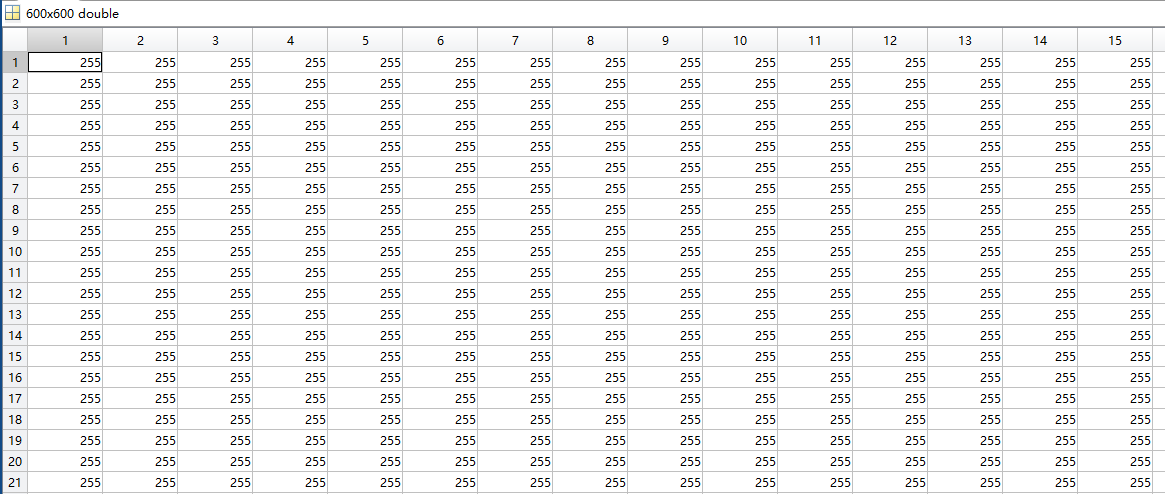• 由于需要frost滤波进行滤波，一通查找到了matlab版本，以前电脑上有matlab软件，但是一直没用到，现在东西好不容易找到了，就搜了下相关教程，整理一个博客。感觉matlab语言和python语言很多类似操作，所以敲起代码...
由于需要frost滤波进行滤波，一通查找到了matlab版本，以前电脑上有matlab软件，但是一直没用到，现在东西好不容易找到了，就搜了下相关教程，整理一个博客。感觉matlab语言和python语言很多类似操作，所以敲起代码来有种“春风得意马蹄疾”的感觉，废话不多说，上代码。下面代码matlab入门没啥问题…算法下载地址如下(如果不需要可以忽略下载，用matlab中自带的算法)：different filters:-Mean filter.-Frost filter.-Modified frost filter(added thresholds).-Lee filter(Using Masks, ENL ect).下载地址：https://download.csdn.net/download/weixin_42727069/123221471-frost滤波使用% 1-frost滤波使用*************************************************clear all;close all;I=im2double(imread('F:\datas\opencvDemoData\560.tiff'));%%I2=im2double(I)%%转换为double类型，避免滤波出错%%BW = dither(I);%通过抖动转换为二值图像来增强图像BW = FrostFilter(I, 7,2.2);%%(I, 4,2.2)% frost滤波使用subplot(121);imshow(I);subplot(122);imshow(BW)2-获取图像信息//2-获取图像信息***********************************clear all;close all;%方法一：info = imfinfo('F:\datas\opencvDemoData\560.tiff','tiff')%直接在命令行显示图片信息%方法二：imtool('F:\datas\opencvDemoData\560.tiff')%弹出窗口显示图片信息3-改变图片格式%%3-改变图片格式***************************************clear all;close all;RGB = imread('F:\datas\opencvDemoData\ren.jpg','jpg');imwrite(RGB,'F:\datas\opencvDemoData\ren.png','png');tupian = imread('F:\datas\opencvDemoData\ren.png');figure;imshow(tupian)4-读取图片中的某一帧%%4-读取图片中的某一帧*********************************clear all;close all;I1 = imread('mri.tif',5);%读取第5帧,mri.tif为matlab自带的样例图片I2 = imread('mri.tif',25);%读取第25帧figure;subplot(121);imshow(I1);subplot(122);imshow(I2);set(gcf,'position',[200,200,500,300]);%% which mri.tif %%查看mri.tif图像位置5-同时显示多帧图像%%5-同时显示多帧图像***************clear all;close all;mri=uint8(zeros(128,128,1,25));for i = 1:25 %25帧[mri(:,:,:,i),map] = imread('mri.tif',i);endmontage(mri,map);%同时显示多帧图像6-将多帧图像转换为电影动图%%6-将多帧图像转换为电影动图*******clear all;close all;mri = uint8(zeros(128,128,1,25));for i =1:25[mri(:,:,:,i),map] = imread('mri.tif',i);endmov = immovie(mri,map);implay(mov);7-图像相加%%7-图像相加，如果大于255 就设置为255*******clear all;close all;I = imread('rice.png');J=imread('cameraman.tif');K = imadd(I,J,'uint16');imshow(K,[]);set(gcf,'position',[400,400,600,600]);8-图像相减%%8-图像相减 ，小于0则设置为0，减去一个常数颜色更深clear all;close all;I = imread('cameraman.tif');J = imsubtract(I,90);imshow(J);9-图像相乘%%9-图像相乘clear all;close all;I = imread('cameraman.tif','tif');J = immultiply(I,0.6);%%乘以小于1的数，图片变暗subplot(121);imshow(I);subplot(122);imshow(J);10-图像的除%%10-图像的除clear all;close all;X = uint8([222,50,21;56,77,89]);Y = uint8([66,66,66;66,66,66]);Z = imdivide(X,Y)11-两幅图像的绝对差异%%11-两幅图像的绝对差异clear all;close all;I = imread('cameraman.tif');J = uint8(filter2(fspecial('gaussian'),I));%对图像进行滤波K = imabsdiff(I,J);%获取滤波图像和之前图像的差异subplot(131);imshow(I);subplot(132);imshow(K);subplot(133);imshow(K,[]);%加[]是为了显示清晰原文链接:https://blog.csdn.net/weixin_42727069/article/details/105456619
展开全文• Matlab是强大的数学问题处理软件，同时也是图像处理强有力的工具。本文通过几个实例具体介绍Matlab下如何进行图像处理。 （1）图像的读取与输出 Matlab读取图像的基本方法是 imageName = imread('nameOfImage');...
Matlab是强大的数学问题处理软件，同时也是图像处理强有力的工具。本文通过几个实例具体介绍Matlab下如何进行图像处理。
（1）图像的读取与输出
Matlab读取图像的基本方法是
imageName = imread('nameOfImage');支持JPG，PNG，BMP和TIF等各种格式的图片，读取后的imageName是一个uint8类型的矩阵，其元素值为图像像素点的灰度。

读取图像后，可用
imshow('imageName');来显示所读取的图像，或者用imwrite(imageName, 'nameOfImage');
将图像输出。
（2）图像的变换
对图像进行变换实际上是通过改变图像各像素点的灰度值以改变图像的视觉效果，一般分为线性和非线性两类。
线性变换实例：image = imread('desert.jpg');

[m,n] = size(image);

min = min(image(:));
max = max(image(:));

for i = 1:m
for j = 1:n
image(i,j) = floor((255 - 0) / (max - min) * (image(i,j) - min) + 0);
end
end

imshow(image);
imwrite(image, 'desert1.jpg');
这样图像就从原来的变为。

（3）图像的空间域平滑与锐化
图像平滑的主要目的是消除噪声或模糊图像，去除小的细节货弥合目标间的缝隙，而图像锐化恰恰相反。方法一般是通过模版与图像的某区域作卷积运算
图像平滑实例：
1.邻域平均法
function outputImage = averageInDomain(image,template)
outputImage = image;
[m, n] = size(image);
for i = 2:(m - 1)
for j = 2:(n - 1)
core = image((i - 1):(i + 1), (j - 1):(j + 1));
temp = 1 / sum(template(:)) * (double(core) .* double(template));
outputImage(i,j) = floor(sum(temp(:)));
end
end

这里template是卷积所用的模版，image是待处理的图像，outputImage是输出的结果。
2.中值滤波法function outputImage = midvalueFilter(image)
[m, n] = size(image);
outputImage = image;
for i = 2:(m - 1)
for j = 2:(n - 1)
core = image((i - 1):(i + 1), (j - 1):(j + 1));
temp = sort(reshape(core, 1, 9));
outputImage(i, j) = temp(5);
end
end

各变量是什么不再赘述。

上面总结了一些常见的图像处理方法，Matlab的功能远比上述所列举的强大。需要不断积累，练习才能较好的掌握Matlab处理图像的技巧。


展开全文实例
• ## Matlab处理图像/视频

千次阅读 2018-04-12 13:12:48
%% MP4文件处理video= VideoReader('video_name.mp4'); %读取MP4文件 nFrames = video.NumberOfFrames; vidHeight = video.Height; vidWidth = video.Width; video_4D=zeros(vidHeight,vidWidth,3,nFrames); % ...
%% MP4文件处理video= VideoReader('video_name.mp4');   %读取MP4文件

nFrames = video.NumberOfFrames;

vidHeight = video.Height;

vidWidth = video.Width;

video_4D=zeros(vidHeight,vidWidth,3,nFrames);  % 3为通道数

for k = 1 : nFrames

imwrite(im, ['original_frame',num2str(k),'.bmp'], 'bmp'); %把im存储成图片，并且存储的文件名根据序号改变

video_4D(:,:,;,k)=im;  %形成4D文件

end

implay（video_4D）  %播放video
展开全文• 在使用Matlab进行图像处理时，发现数据一超过255就会溢出，最大就是255。 出错原因 Matlab中读取图片后保存的数据是uint8类型(取值范围0~255)，但在图像矩阵运算的时候，使用的数据类型却是double类型。若使用uint8...
错误描述
在使用Matlab进行图像处理时，发现数据一超过255就会溢出，最大就是255。出错原因
Matlab中读取图片后保存的数据是uint8类型(取值范围0~255)，但在图像矩阵运算的时候，使用的数据类型却是double类型。若使用uint8类型的数据进行矩阵运算，一旦数据超出255就会溢出。
解决方法

将读入的图像数据转化为double类型 ，转换结果取值I位于[0,255]。【推荐】
使用im2double() 进行转换，转换结果取值I位于[0,1]。
此时，图像处理完成后，要使用im2uint8()或uint8(round(I*255)) 将图像数据的灰度值范围变回[0,255]。
将读入的图像数据转化为uint16类型，转换结果取值I位于[0,255]。

使用double类型的好处有以下两点：
①部分函数只支持double类型。
②保证图像数据运算的精度，防止数据溢出。


展开全文uint8
• MATLAB在写m文件时，语句后面如果加；，语句运行，但不会在命令窗口（command windows）输出结果，如果不加分号，即运算，又会在命令窗口输出结果。 2. size（）：获取矩阵的行数和列数 （1）s=size(A), ...
• A=imread('pyy.jpg'); %读取照片 A=double(A); %是矩阵变为数值型 A(:,1:100)=0; %使得边缘的100行变为0，从而达到边框效果 A(:,1900:2000)=0; A(1:200,:)=0; A(2793:2993,:)=0; A=uint8(A);......
• MATLAB图像处理MATLAB图像处理MATLAB图像MATLAB图像处理
• 因为c是uint8类型的，所以一定要注意这一点，在matlab中用whos命令查阅一下c, 便有： Name Size Bytes Class Attributes c 1x1 1 uint8 所以，上面的程序应该改为： clear clc cd('C:\Documents and ...
• MATLAB图形图像处理——图像灰度变换，介绍matlab处理图像的基础知识、图像灰度变换的基础知识。 通过直方图均衡化改变图像对比度和亮度，将模糊的图像变清晰。计算机视觉
• ## MATLAB图像处理

千人学习 2017-06-27 19:37:11
• ## matlab图像处理

热门讨论 2009-05-27 10:52:56
matlab图像处理 matlab图像处理 matlab图像处理 matlab图像处理 matlab图像处理
• ## 图像处理之Matlab图像读取

万次阅读 多人点赞 2017-05-29 12:50:45
• 利用MATLAB写的，关于医学图像处理的代码，将图像处理技术应用于医学领域。利用MATLAB写的，关于医学图像处理的代码，将图像处理技术应用于医学领域。
• 计算机图像处理实验课1-9，看完本课程可以让你matlab技能小小进步，对图像处理稍微了解。、 （一）matlab数字图像处理实验-guide的简单使用教程 （二）matlab数字图像处理实验-图像的几何变换 （三）matlab数字...
• ## MATLAB图形图像处理

热门讨论 2011-01-03 12:20:11
8.6 MATLAB 中的 8 位和 16 位图像 8.6.1 8 位和 16 位索引图像 8.6.2 8 位和 16 位灰度图像 8.6.3 8 位和 16 位 RGB 图像 8.7 图像文件的操作 8.7.1 查询图像文件的信息 8.7.2 图像文件的读取 8.7.3 图像...
• 转自：...MATLAB还支持另一种类型无符号整型(uint8)，即图像矩阵中每个数据占用1个字节。在使用MATLAB工具箱时，一定要注意函数所要求的参数类型。另外，uint8与double两种...
• 昨天给大家分享了最新版本的MATLAB软件，大家给的反馈还不错，蓝熙就想着既然大家都需要MATLAB，那么光有软件还不行，还得会用呀。所以今天蓝熙就给大家带来一套非常好用的MATLAB视频教程，虽然以前蓝熙也给大家分享...
• MATLAB彩色图像处理 （ps:这篇文章里面用到数字图像处理的工具包，若需要请留言） 一、对彩色图像的表达和显示 %%对彩色图像的表达和显示 %%A.显示彩色立方体 figure,rgbcube(0,0,10);%从正面观察彩色立方体 figure,...算法
• 本文介绍了MATLAB 语言的特点,基于MATLAB的数字图像处理环境，介绍了如何利用MATLAB及其图像处理工具箱进行数字图像处理，并通过一些例子来说明利用MATLAB图像处理工具箱进行图像处理的方法。主要论述了利用MATLAB...
• 写在前头：说到数字图像处理，不得不提起MATLAB。这是一款非常方便的仿真软件，绝大多数的图像处理可以用MATLAB完成。 　有人问，处理图片，用PS岂不是更好。两者各有优点，如果需要将1００００幅图片转换成灰度...软件 图片
• 吃小羊：MATLAB图像处理：01：基本图像导入，处理和导出​zhuanlan.zhihu.com吃小羊：MATLAB图像处理：02：检测和测量图像中的圆形物体​zhuanlan.zhihu.com吃小羊：MATLAB图像处理：03：纠正照明不均匀并分析前景...
• 三维点云图像处理步骤 Matlab函数库 https://ww2.mathworks.cn/help/vision/lidar-and-point-cloud-processing.html?s_tid=CRUX_lftnavhtml
• 图像复原技术 图像在采集、传送、转换过程中会混入...在Matlab中，函数 imnoise 可以给图像加入高斯噪声、椒盐噪声、泊松分布噪声和乘性噪声 二、空域内的滤波复原方法 1、均值滤波 (1)、算术均值滤波 令 Sxy 表示......

# matlab处理图像matlab 订阅